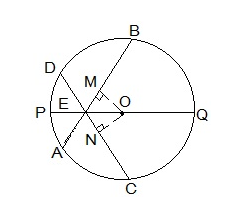Guru

# If two equal chords of a circle intersect within the circle, prove that the line joining the point of intersection to the centre makes equal angles with the chords. Q.3

• 0

Please guide me the best way for solving the question of class 9th math of Circles chapter of exercise 10.4 of math of question no.(3) What is the best way for solving this question, please guide me the best way If two equal chords of a circle intersect within the circle, prove that the line joining the point of intersection to the centre makes equal angles with the chords.

Share

1. From the question we know the following:

(i) AB and CD are 2 chords which are intersecting at point E.

(ii) PQ is the diameter of the circle.

(iii) AB = CD.

Now, we will have to prove that BEQ = CEQ

For this, the following construction has to be done:

Construction:

Draw two perpendiculars are drawn as OM ⊥ AB and ON ⊥ D. Now, join OE. The constructed diagram will look as follows:Now, consider the triangles ΔOEM and ΔOEN.

Here,

(i) OM = ON [Since the equal chords are always equidistant from the centre]

(ii) OE = OE [It is the common side]

(iii) OME = ONE [These are the perpendiculars]

So, by RHS congruency criterion, ΔOEM ΔOEN.

Hence, by CPCT rule, MEO = NEO

∴ BEQ = CEQ (Hence proved).

• 0